Writing Fractions as Decimals worksheet

Chapter 8 Class 6 Decimals
Serial order wise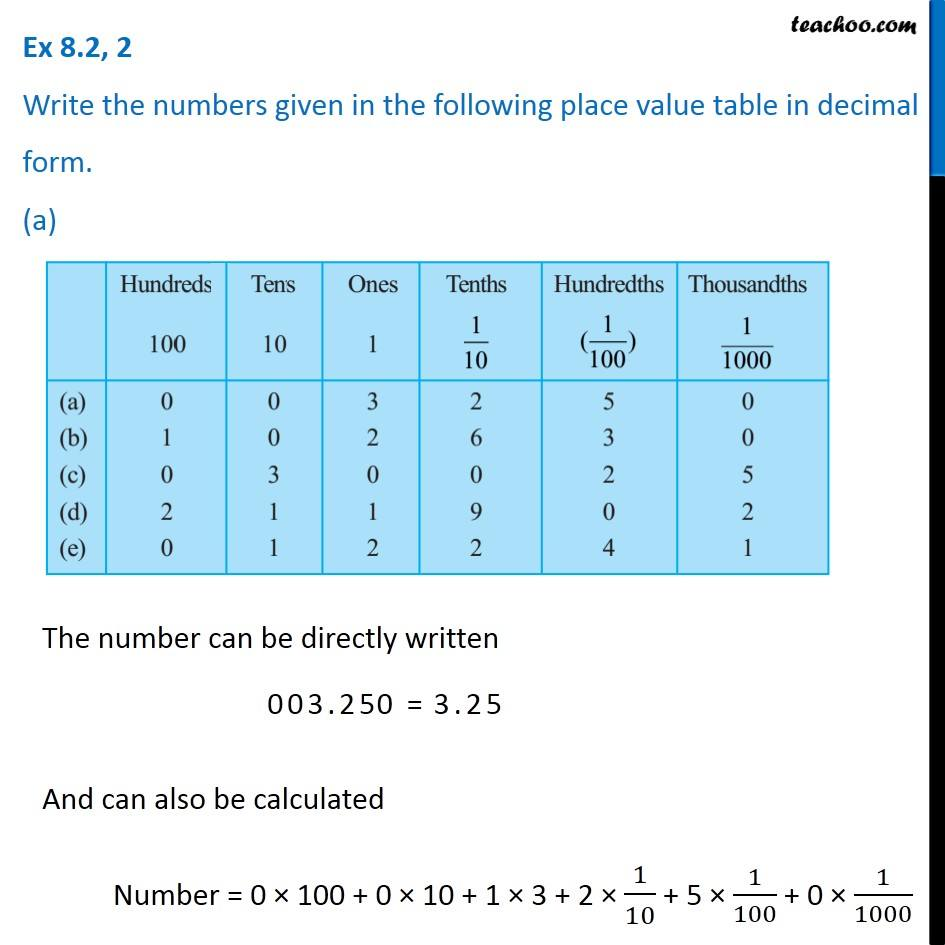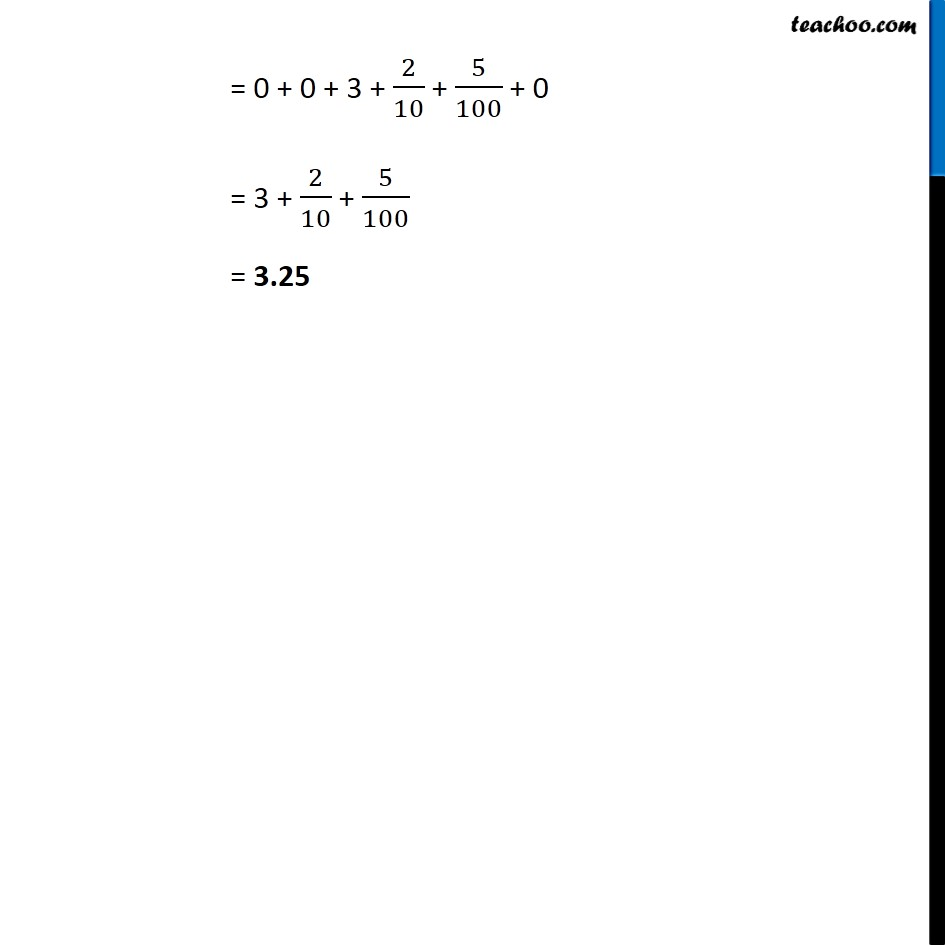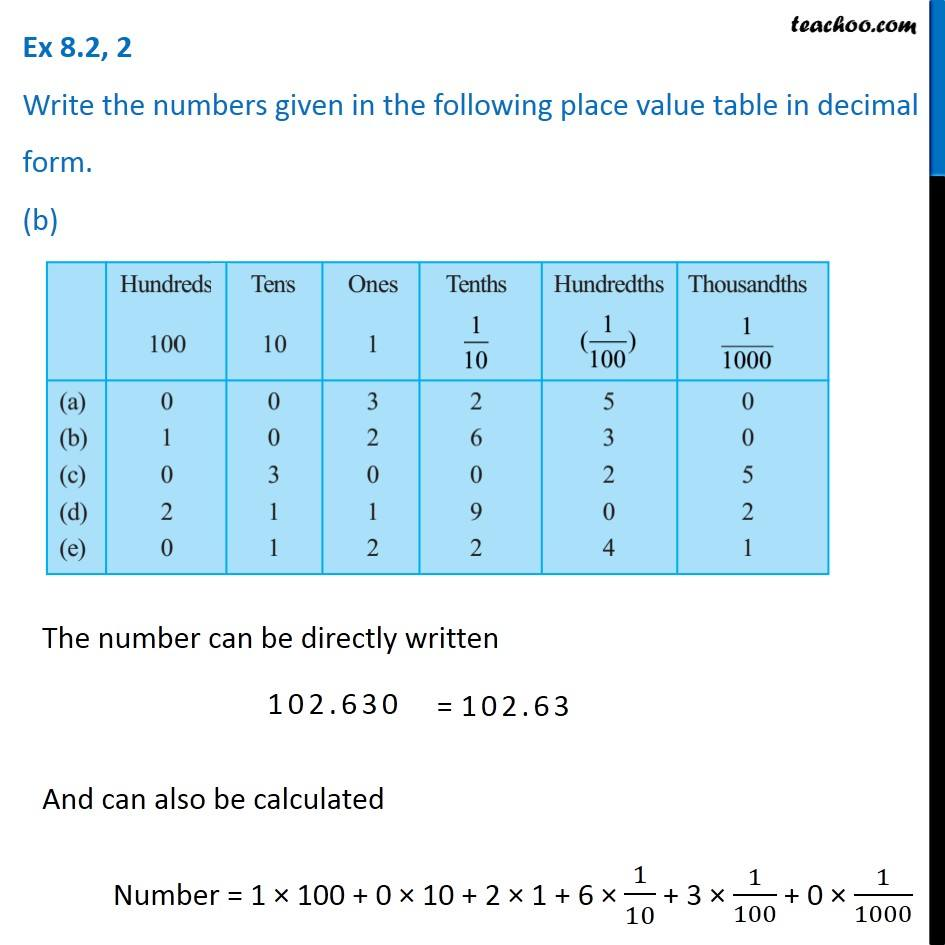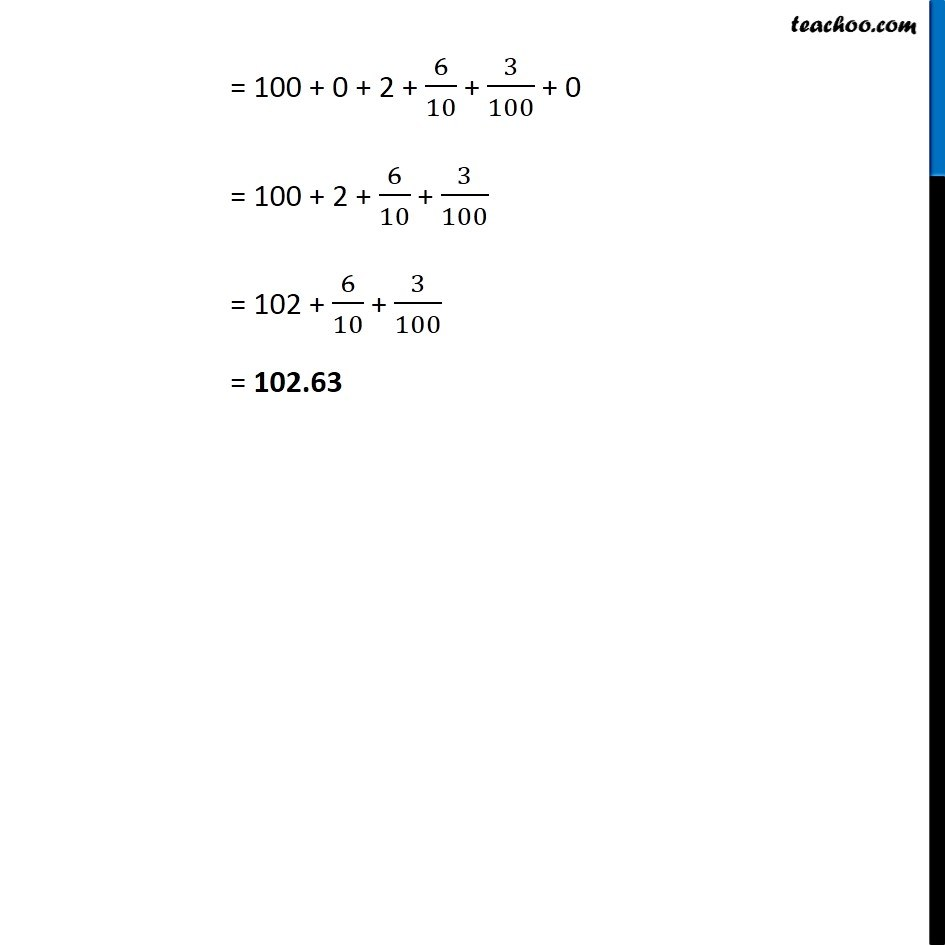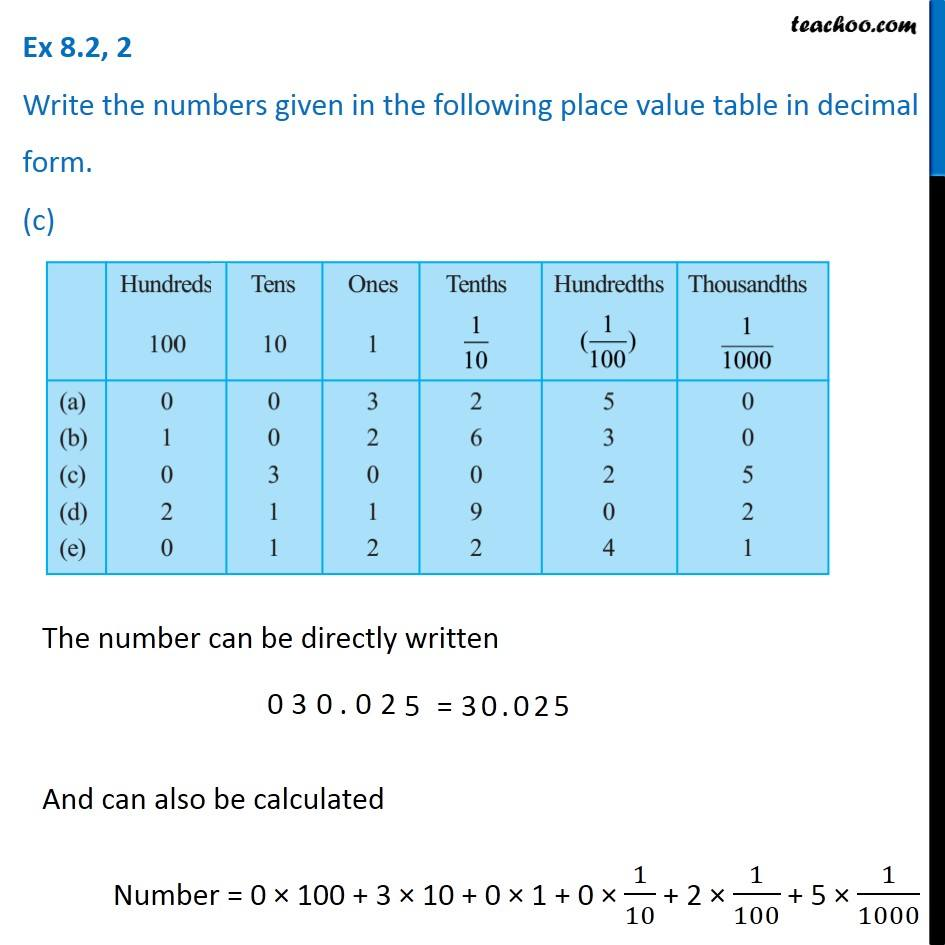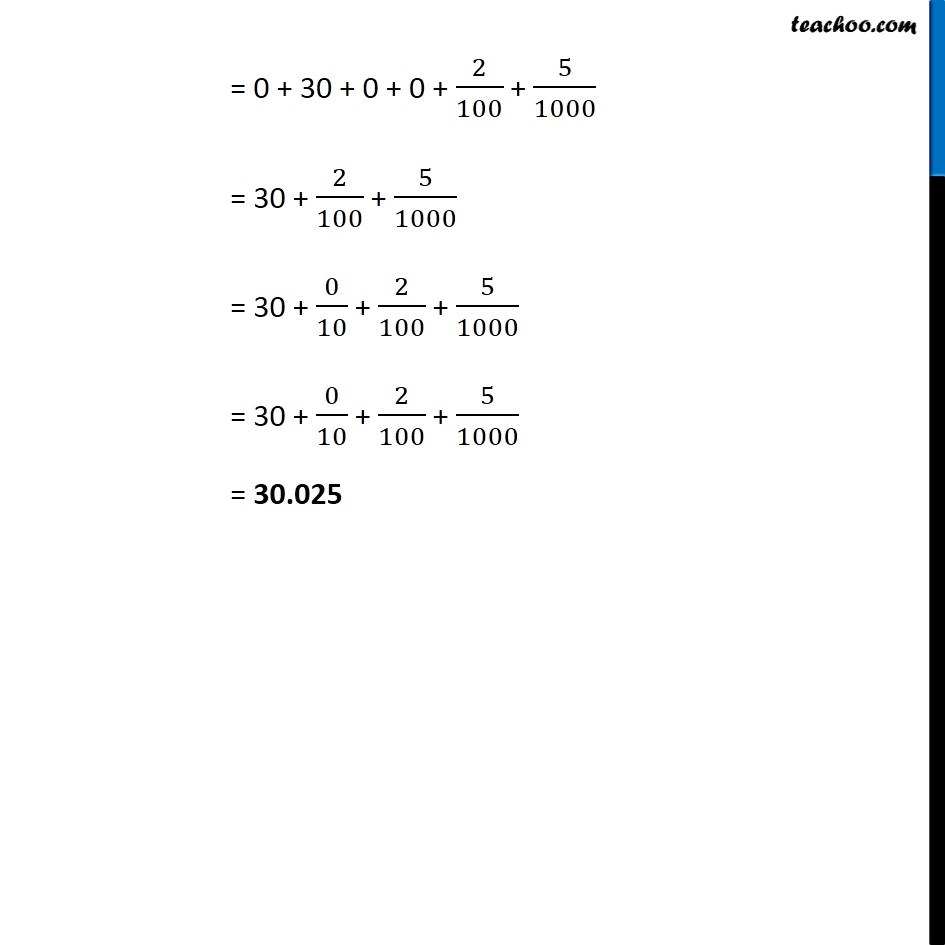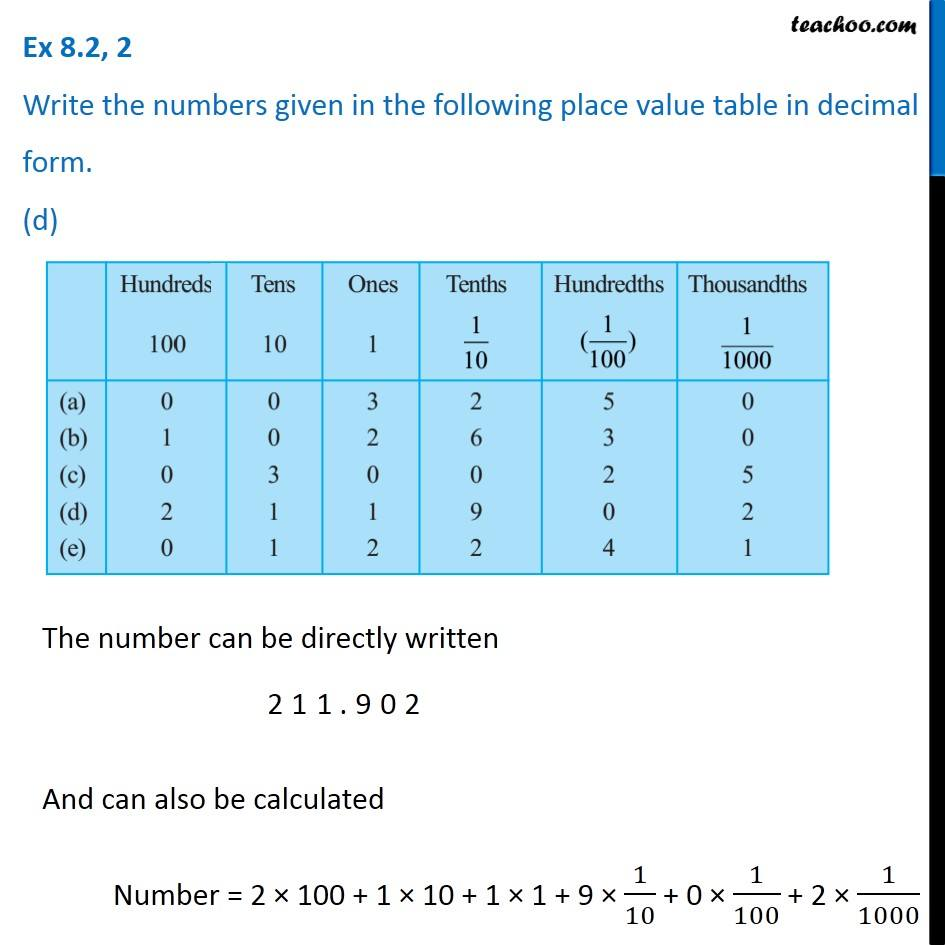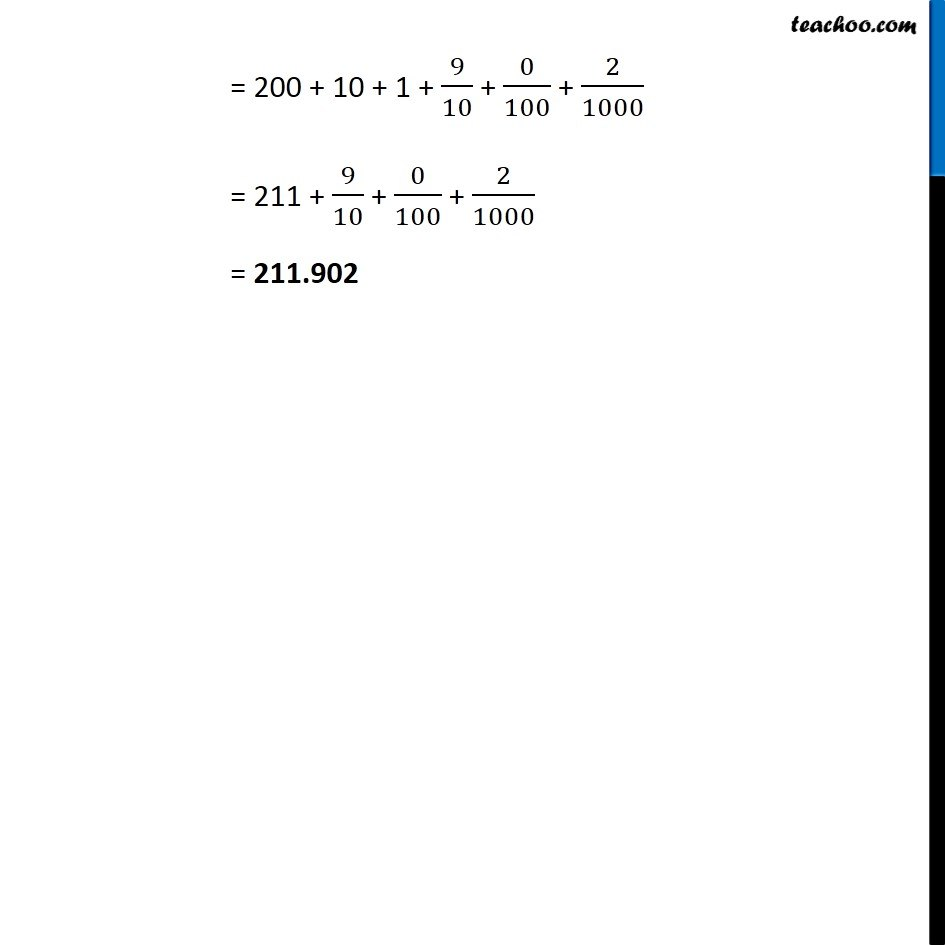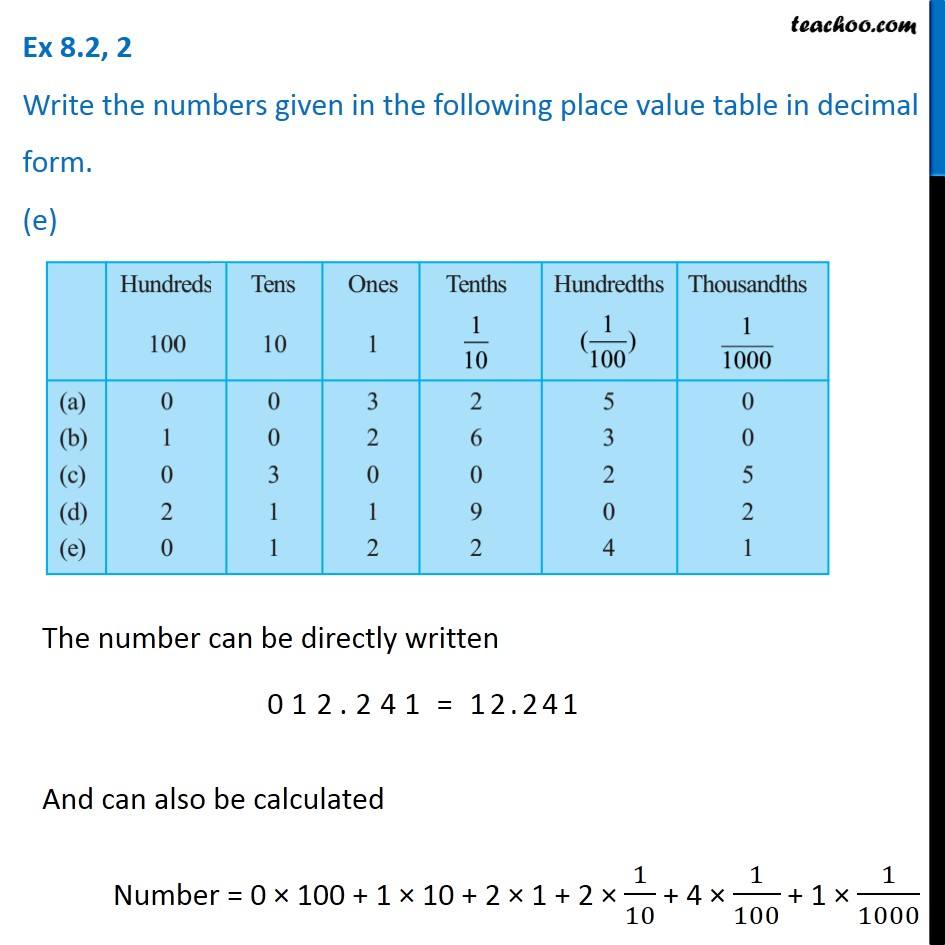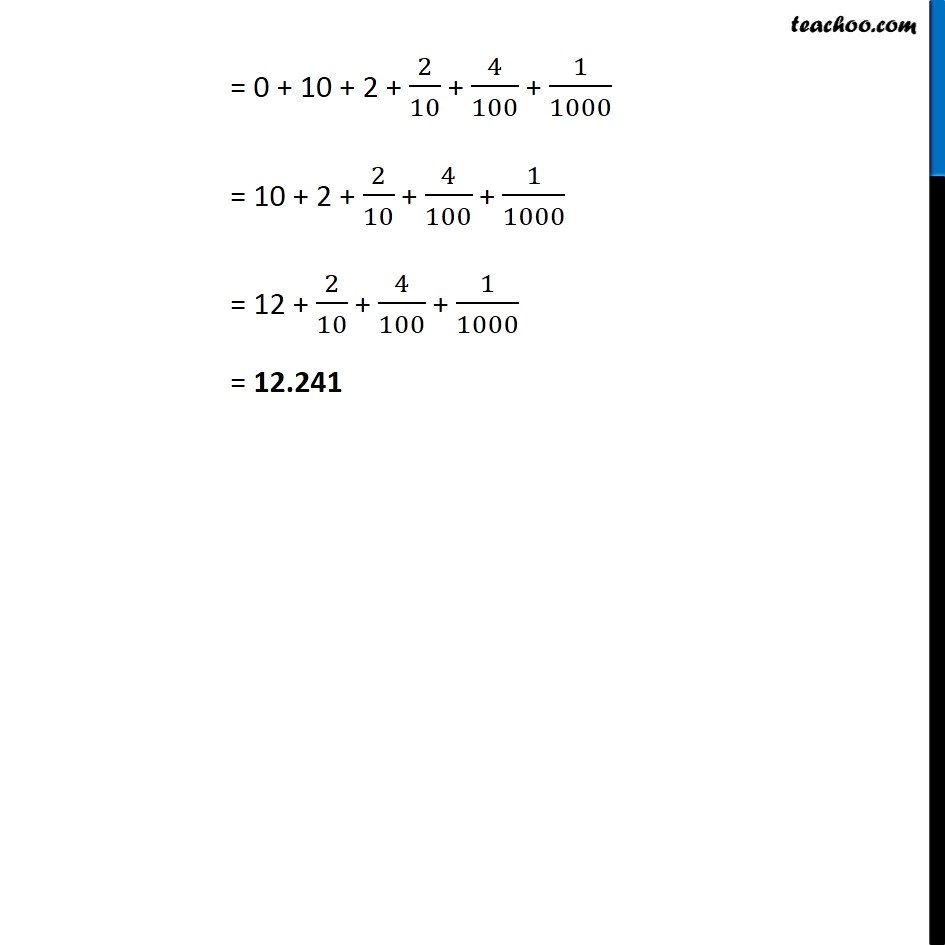Learn in your speed, with individual attention - Teachoo Maths 1-on-1 Class

### Transcript

Question 2 Write the numbers given in the following place value table in decimal form. (a) The number can be directly written And can also be calculated Number = 0 100 + 0 10 + 1 3 + 2 1/10 + 5 1/100 + 0 1/1000 = 0 + 0 + 3 + 2/10 + 5/100 + 0 = 3 + 2/10 + 5/100 = 3.25 Question 2 Write the numbers given in the following place value table in decimal form. (b) The number can be directly written And can also be calculated Number = 1 100 + 0 10 + 2 1 + 6 1/10 + 3 1/100 + 0 1/1000 = 100 + 0 + 2 + 6/10 + 3/100 + 0 = 100 + 2 + 6/10 + 3/100 = 102 + 6/10 + 3/100 = 102.63 Question 2 Write the numbers given in the following place value table in decimal form. (c) The number can be directly written And can also be calculated Number = 0 100 + 3 10 + 0 1 + 0 1/10 + 2 1/100 + 5 1/1000 = 0 + 30 + 0 + 0 + 2/100 + 5/1000 = 30 + 2/100 + 5/1000 = 30 + 0/10 + 2/100 + 5/1000 = 30 + 0/10 + 2/100 + 5/1000 = 30.025 Question 2 Write the numbers given in the following place value table in decimal form. (d) The number can be directly written And can also be calculated Number = 2 100 + 1 10 + 1 1 + 9 1/10 + 0 1/100 + 2 1/1000 = 200 + 10 + 1 + 9/10 + 0/100 + 2/1000 = 211 + 9/10 + 0/100 + 2/1000 = 211.902 Question 2 Write the numbers given in the following place value table in decimal form. (e) The number can be directly written And can also be calculated Number = 0 100 + 1 10 + 2 1 + 2 1/10 + 4 1/100 + 1 1/1000 = 0 + 10 + 2 + 2/10 + 4/100 + 1/1000 = 10 + 2 + 2/10 + 4/100 + 1/1000 = 12 + 2/10 + 4/100 + 1/1000 = 12.241